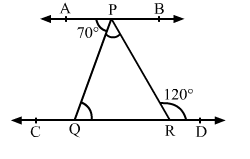# In the given figure, AB || CD. If ∠APQ = 70° and ∠PRD = 120°, then ∠QPR = ?

Question:

In the given figure, AB || CD. If APQ = 70° and ∠PRD = 120°, then ∠QPR = ?
(a) 50°
(b) 60°
(c) 40°
(d) 35°Solution:

(a) 50°

$A B \| C D$ and $P Q$ is the transversal.

$\therefore \angle P Q R=\angle A P Q=70^{\circ} \quad$ [Alternate Interior Angles]

Side QR of traingle PQR is produced to D.

$\therefore \angle P Q R+\angle Q P R=\angle P R D$

$\Rightarrow 70^{\circ}+\angle Q P R=120^{\circ}$

$\Rightarrow \angle Q P R=50^{\circ}$